User’s Guide : Estimation and Solution Options : Nonlinear Equation Solution Methods

Nonlinear Equation Solution Methods
When solving a nonlinear equation system, EViews first analyzes the system to determine if the system can be separated into two or more blocks of equations which can be solved sequentially rather than simultaneously. Technically, this is done by using a graph representation of the equation system where each variable is a vertex and each equation provides a set of edges. A well known algorithm from graph theory is then used to find the strongly connected components of the directed graph.
Once the blocks have been determined, each block is solved for in turn. If the block contains no simultaneity, each equation in the block is simply evaluated once to obtain values for each of the variables.
If a block contains simultaneity, the equations in that block are solved by either a Gauss-Seidel or Newton method, depending on how the solver options have been set.
Gauss-Seidel
By default, EViews uses the Gauss-Seidel method when solving systems of nonlinear equations. Suppose the system of equations is given by: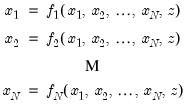(59.16)
where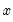are the endogenous variables and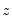are the exogenous variables.
The problem is to find a fixed point such that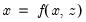. Gauss-Seidel employs an iterative updating rule of the form: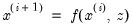(59.17)
to find the solution. At each iteration, EViews solves the equations in the order that they appear in the model. If an endogenous variable that has already been solved for in that iteration appears later in some other equation, EViews uses the value as solved in that iteration. For example, the k-th variable in the i-th iteration is solved by: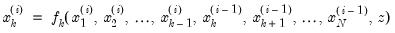(59.18)
The performance of the Gauss-Seidel method can be affected be reordering of the equations. If the Gauss-Seidel method converges slowly or fails to converge, you should try moving the equations with relatively few and unimportant right-hand side endogenous variables so that they appear early in the model.
Newton's Method
Newton’s method for solving a system of nonlinear equations consists of repeatedly solving a local linear approximation to the system.
Consider the system of equations written in implicit form: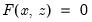(59.19)
where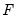is the set of equations,is the vector of endogenous variables andis the vector of exogenous variables.
In Newton’s method, we take a linear approximation to the system around some values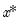and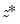: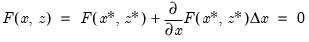(59.20)
and then use this approximation to construct an iterative procedure for updating our current guess for: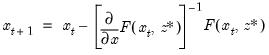(59.21)
where raising to the power of -1 denotes matrix inversion.
The procedure is repeated until the changes inbetween periods are smaller than a specified tolerance.
Note that in contrast to Gauss-Seidel, the ordering of equations under Newton does not affect the rate of convergence of the algorithm.
Broyden's Method
Broyden's Method is a modification of Newton's method which tries to decrease the calculational cost of each iteration by using an approximation to the derivatives of the equation system rather than the true derivatives of the equation system when calculating the Newton step. That is, at each iteration, Broyden's method takes a step: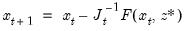(59.22)
where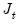is the current approximation to the matrix of derivatives of the equation system.
As well as updating the value ofat each iteration, Broyden's method also updates the existing Jacobian approximation,, at each iteration based on the difference between the observed change in the residuals of the equation system and the change in the residuals predicted by a linear approximation to the equation system based on the current Jacobian approximation.
In particular, Broyden's method uses the following equation to update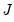: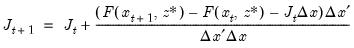(59.23)
where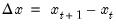. This update has a number of desirable properties (see Chapter 8 of Dennis and Schnabel (1983) for details).
In EViews, the Jacobian approximation is initialized by taking the true derivatives of the equation system at the starting values of. The updating procedure given above is repeated until changes inbetween periods become smaller than a specified tolerance. In some cases the method may stall before reaching a solution, in which case a fresh set of derivatives of the equation system is taken at the current values of, and the updating is continued using these derivatives as the new Jacobian approximation.
Broyden's method shares many of the properties of Newton's method including the fact that it is not dependent on the ordering of equations in the system and that it will generally converge quickly in the vicinity of a solution. In comparison to Newton's method, Broyden's method will typically take less time to perform each iteration, but may take more iterations to converge to a solution. In most cases Broyden's method will take less overall time to solve a system than Newton's method, but the relative performance will depend on the structure of the derivatives of the equation system.